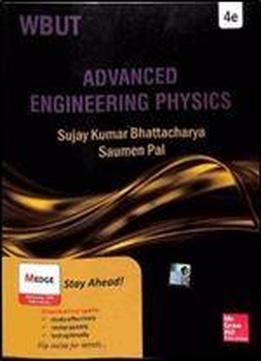# Adv Engineering Physics (wbut 2014)Tags: Various

Aim of this Book The West Bengal University of Technology recently reorganized the physics syllabus for its BTech program. It has divided the entire syllabus into two parts. Topics to be studied by the students of both physics-based and chemistry-based streams of engineering have been compiled as the first part and have been made compulsory for all first-year students. Some other topics have been put under the second part, which are to be studied as part of the compulsory curriculum by the second-year students of physics-based streams only. We have compiled the following topics of the second part of the BTech syllabus in this book: (a) vector analysis, (b) electrostatics, © dielectrics, (d) magnetostatics, (e) electromagnetic field theory, (f) classical mechanics, (g) quantum mechanics and (h) statistical mechanics. The book is, hence, appropriately titled, Advanced Engineering Physics. This book consists of eight chapters. Chapter 1 explains vectors and discusses algebra and calculus along with gradient of scalar field, divergence of vector field and curl of a vector. Chapter 2 elucidates electrostatics. Under electrostatics, quantisation of charge, conservation of charge, Coulomb’s law, electric field, electric potential, electric flux, Gauss’ law and Poisson’s and Laplace’s equations have been discussed. Chapter 3 presents discussions of dielectrics. Under this topic, electric polarizations, polarizability and polarization in monatomic and polyatomic gases are also described. Chapter 4 elucidates magnetostatics. Biot–Savart Law, Ampere’s circuital law and magnetic scalar and vector potentials. Chapter 5 discusses electromagnetic field theory. Maxwell’s equations in both differential and integral forms are included here. Chapter 6 discusses topics on classical mechanics elaborate such as constraints of motion, generalized coordinates, degrees of freedom, D’ Alembert’s principle, lagrangian formulation, hamiltonian formulation, etc. Chapter 7 discusses quantum mechanics, especially wave function representing a particle in space, operators in quantum mechanics and development of Schrodinger’s wave equation. Chapter 8 elucidates statistical mechanics. Bose–Einstein and Fermi–Dirac statistics are also discussed along with a comparative study of the three statistical distribution functions.Preface xiii Appendix A and Appendix B explain surface integrals and angular concept, respectively. Appendix C provides a list of useful physical constants. Appendix D elucidates curl of gradient, divergence, curl and Laplacian. A set of 11 experiments in proper format, with required aims, theories, tables and procedures are included in the book as Appendix E. This section covers the syllabus of the practical classes for this course. Hence, the students will no longer require a separate book for their practical classes. Review exercises are divided into three parts. Part 1 comprises multiple choice questions and short questions with answers. Part 2 consists of descriptive questions and Part 3 comprises unsolved problems under the heading numerical problems. For solving such problems, students will have to apply critically, concepts which they have already learnt in the text.